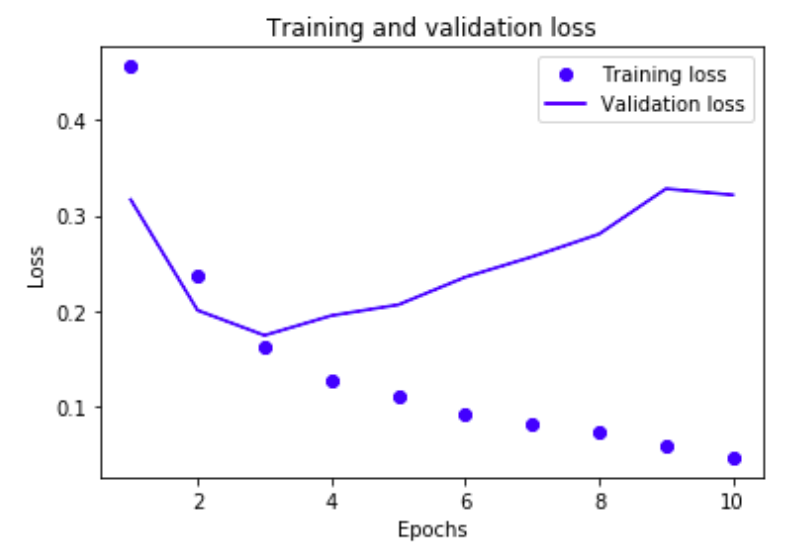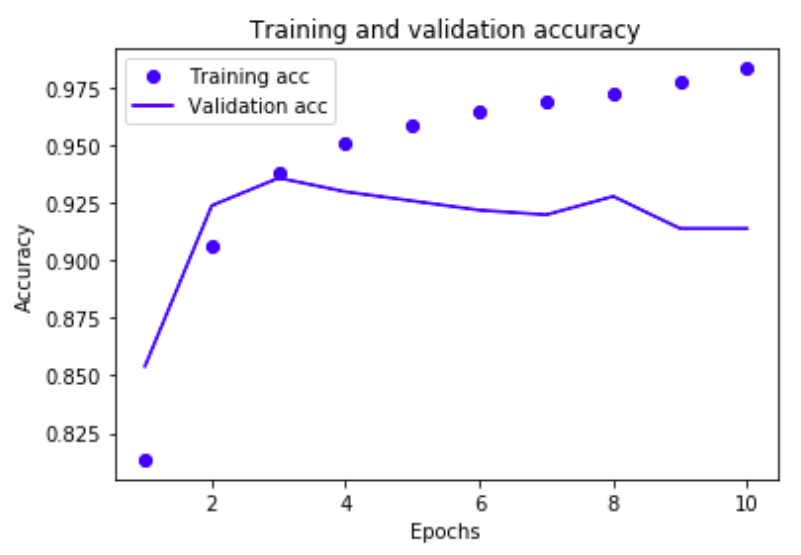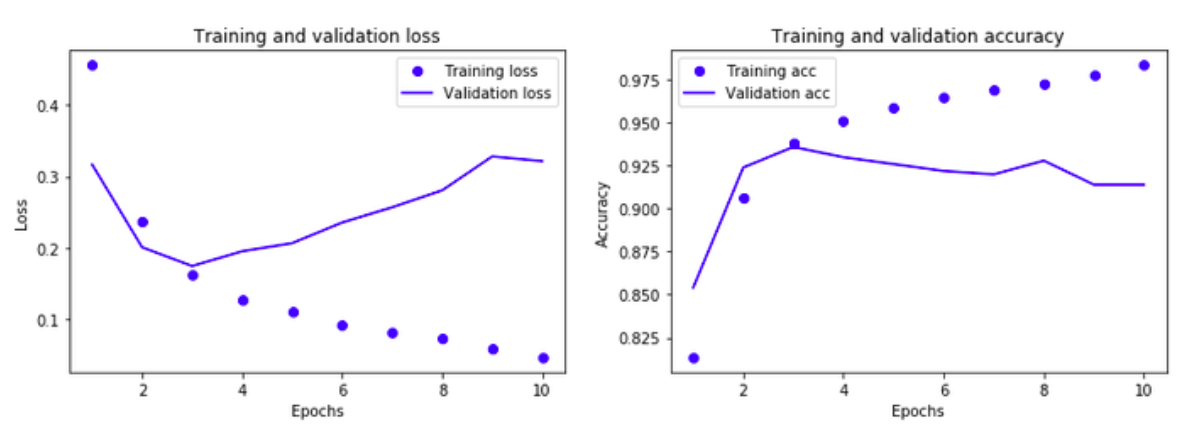Over a million developers have joined DZone.{{announcement.body}}
{{announcement.title}}

Sentiment Analysis on US Airline Twitters Dataset: A Deep Learning Approach

DZone 's Guide to

Sentiment Analysis on US Airline Twitters Dataset: A Deep Learning Approach

Learn about using deep learning, neural networks, and classification with TensorFlow and Keras to analyze the Twitter accounts of US airlines.

· AI Zone ·
Free Resource

Comment (0)

Save
{{ articles.views | formatCount}} Views

In two of my previous posts (this and this), I tried to do sentiment analysis on the Twitter airline dataset with one of the classic machine learning techniques: Naive-Bayesian classifiers. For this post, I did one classifier with a deep learning approach. This work won't be seminal; it's only an expedient to play a little bit with neural networks.

For this work, I used Tensorflow and Keras to define the neural network and the new Jupyter Lab to write the code (I think it's really cool!). If you would, you can find my data science environment, with all of this stuff dockerized at this link.

OK, now, let's talk about the neural network used in this post. The most interesting layer is the LSTM layer. If you want to know more about LSTM, I suggest to the read this post from Christopher Olah's blog. LSTM layers are widely used for language processing, which is why I used this kind of layer for my analysis. A schema of the very simple neural network for this example if the following:The entire notebook used for this analysis is just down here and can be found on my GitHub profile here. Every code block is commented, so I don't want to annoy you with a lot of words, let's let the code talk...

In :

``````import keras
from keras.modelsimportSequential
from keras.layersimportDense, Dropout, Embedding, LSTM, SpatialDropout1D
from keras.callbacksimportModelCheckpoint
import os
from sklearn.metricsimportroc_auc_score
import matplotlib.pyplotasplt
import pandasaspd
import numpyasnp
import re
from keras.preprocessing.textimportTokenizer
from sklearn.model_selectionimporttrain_test_split``````

In :

``tweets=pd.read_csv('./Dataset/Tweets.csv',sep=',')``

In :

``tweets.head(2)``

Out :

To view this table, scroll down to the appropriate section in this article.

Select only interesting fields.

In :

``data = tweets[['text','airline_sentiment']]``

Clean up the dataset, considering only positive and negative tweets.

In :

``````data = data[data.airline_sentiment != "neutral"]
data['text'] = data['text'].apply(lambda x: x.lower())
data['text'] = data['text'].apply((lambda x: re.sub('[^a-zA-z0-9\s]','',x)))

print(data[ data['airline_sentiment'] == 'positive'].size)
print(data[ data['airline_sentiment'] == 'negative'].size)``````
``````4726
18356``````

Tokenization

In :

``````max_fatures = 2000
tokenizer = Tokenizer(num_words=max_fatures, split=' ')
tokenizer.fit_on_texts(data['text'].values)
X = tokenizer.texts_to_sequences(data['text'].values)

Neural Network

In :

``````embed_dim = 128
lstm_out = 196

model = Sequential()
model.compile(loss = 'categorical_crossentropy', optimizer='adam',metrics = ['accuracy'])
print(model.summary())``````
``````_________________________________________________________________
Layer (type)                 Output Shape              Param #
=================================================================
embedding_5 (Embedding)      (None, 32, 128)           256000
_________________________________________________________________
dropout_2 (Dropout)          (None, 32, 128)           0
_________________________________________________________________
lstm_4 (LSTM)                (None, 196)               254800
_________________________________________________________________
dense_4 (Dense)              (None, 2)                 394
=================================================================
Total params: 511,194
Trainable params: 511,194
Non-trainable params: 0
_________________________________________________________________
None``````

Declaring Dataset

In :

``````Y = pd.get_dummies(data['airline_sentiment']).values
X_train, X_test, Y_train, Y_test = train_test_split(X,Y, test_size = 0.33, random_state = 42)
print(X_train.shape,Y_train.shape)
print(X_test.shape,Y_test.shape)``````
``````(7732, 32) (7732, 2)
(3809, 32) (3809, 2)``````

Selecting Some Data for Training and Some for Validation

In :

``````X_val = X_train[:500]
Y_val = Y_train[:500]``````

In :

``````partial_X_train = X_train[500:]
partial_Y_train = Y_train[500:]``````

Train the Network

In :

``````batch_size = 512
history = model.fit(partial_X_train,
partial_Y_train,
epochs = 10,
batch_size=batch_size,
validation_data=(X_val, Y_val))``````
``````Train on 7232 samples, validate on 500 samples
Epoch 1/10
7232/7232 [==============================] - 21s 3ms/step - loss: 0.5730 - acc: 0.7688 - val_loss: 0.4338 - val_acc: 0.7960
Epoch 2/10
7232/7232 [==============================] - 20s 3ms/step - loss: 0.4080 - acc: 0.8191 - val_loss: 0.3696 - val_acc: 0.8340
Epoch 3/10
7232/7232 [==============================] - 21s 3ms/step - loss: 0.3306 - acc: 0.8550 - val_loss: 0.2776 - val_acc: 0.8800
Epoch 4/10
7232/7232 [==============================] - 20s 3ms/step - loss: 0.2467 - acc: 0.8993 - val_loss: 0.2147 - val_acc: 0.9140
Epoch 5/10
7232/7232 [==============================] - 20s 3ms/step - loss: 0.1885 - acc: 0.9266 - val_loss: 0.1954 - val_acc: 0.9300
Epoch 6/10
7232/7232 [==============================] - 20s 3ms/step - loss: 0.1549 - acc: 0.9382 - val_loss: 0.1780 - val_acc: 0.9380
Epoch 7/10
7232/7232 [==============================] - 20s 3ms/step - loss: 0.1410 - acc: 0.9484 - val_loss: 0.1842 - val_acc: 0.9380
Epoch 8/10
7232/7232 [==============================] - 20s 3ms/step - loss: 0.1231 - acc: 0.9537 - val_loss: 0.1877 - val_acc: 0.9300
Epoch 9/10
7232/7232 [==============================] - 20s 3ms/step - loss: 0.1121 - acc: 0.9588 - val_loss: 0.1923 - val_acc: 0.9280
Epoch 10/10
7232/7232 [==============================] - 20s 3ms/step - loss: 0.1024 - acc: 0.9599 - val_loss: 0.1984 - val_acc: 0.9280``````

In :

``````import matplotlib.pyplot as plt
loss = history.history['loss']
val_loss = history.history['val_loss']
epochs = range(1, len(loss) + 1)
plt.plot(epochs, loss, 'bo', label='Training loss')
plt.plot(epochs, val_loss, 'b', label='Validation loss')
plt.title('Training and validation loss')
plt.xlabel('Epochs')
plt.ylabel('Loss')
plt.legend()
plt.show()``````In :

``````plt.clf()
acc = history.history['acc']
val_acc = history.history['val_acc']
plt.plot(epochs, acc, 'bo', label='Training acc')
plt.plot(epochs, val_acc, 'b', label='Validation acc')
plt.title('Training and validation accuracy')
plt.xlabel('Epochs')
plt.ylabel('Accuracy')
plt.legend()
plt.show()``````Validation

In :

``````pos_cnt, neg_cnt, pos_correct, neg_correct = 0, 0, 0, 0
for x in range(len(X_validate)):

result = model.predict(X_validate[x].reshape(1,X_test.shape),batch_size=1,verbose = 2)

if np.argmax(result) == np.argmax(Y_validate[x]):
if np.argmax(Y_validate[x]) == 0:
neg_correct += 1
else:
pos_correct += 1

if np.argmax(Y_validate[x]) == 0:
neg_cnt += 1
else:
pos_cnt += 1

print("pos_acc", pos_correct/pos_cnt*100, "%")
print("neg_acc", neg_correct/neg_cnt*100, "%")``````
``````pos_acc 78.26086956521739 %
neg_acc 94.44444444444444 %``````

Conclusion

To train this network, I used my dockerized data science environment on my laptop without any kind of GPU in a few minutes.

As we can see from the graphs Training and validation loss and Training and validation accuracy, the 3rd epoch is the best before the network start to overfitting the data.The accuracy of prediction with this network jumps from 86% to 94%, compared to the previous Naive-Bayesian classifiers with a very simple network and few epochs. The accuracy of the positive tweets is increased, too. Despite the accuracy increase with this kind of network, I think the accuracy can be improved, and this is the goal of my next tests.

Please feel free to comment and contact me to discuss this post!

Topics:
machine learning ,neural network ,sentiment analysis ,deep learning ,ai ,twitter ,tutorial ,classification ,tensorflow ,keras

Comment (0)

Save
{{ articles.views | formatCount}} Views

Published at DZone with permission of

Opinions expressed by DZone contributors are their own.